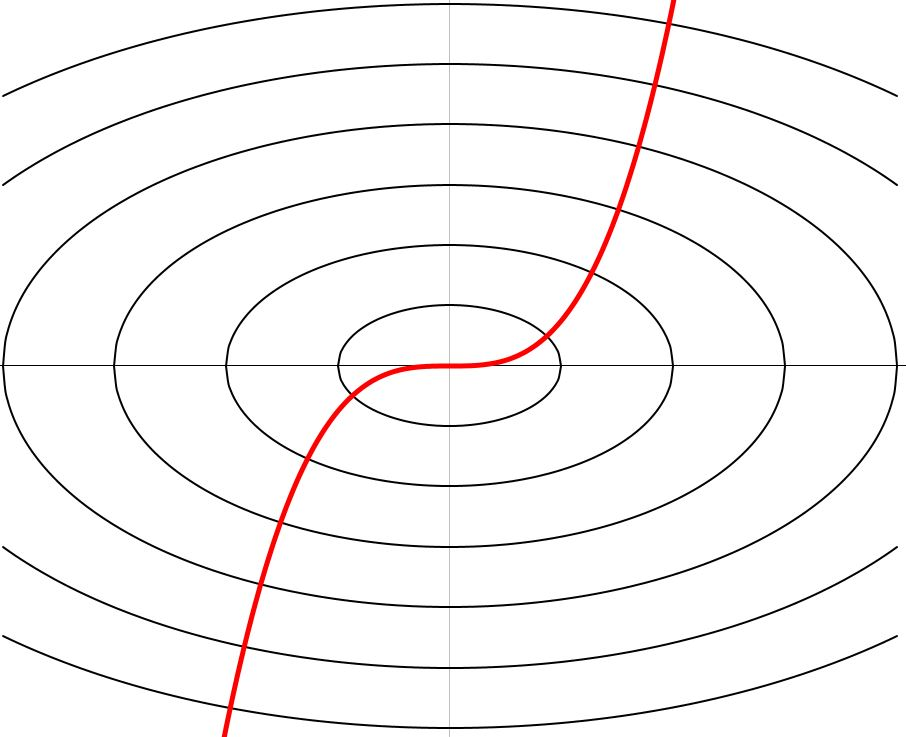# Elliptic Curve?

Calculus Level 3Consider the family of ellipses $E_r:\ x^2 + ay^2 = r^2\ \ \ \ \ r > 0.$ If the graph of $y = x^3$ intersects all $E_r$ perpendicularly, how much is $a$?

×